Next: Gustavo Granja - On HPn Up: 2)  Homotopy Theory / Théorie Previous: Dan Christensen - Phantom

## F. R. Cohen - On stunted projective spaces

 F. R. COHEN, Department of Mathematics, University of Rochester, Rochester, New York  14627, USA On stunted projective spaces

F. Sergeraert and V. Smirnov have recently studied the homology of the loop spaces for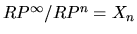. It is the purpose of this note to record the homotopy type of these spaces after looping (sometimes more than once). The following is joint work with R. Levi.

Theorem. (1)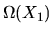is homotopy equivalent to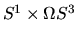.
(2)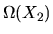is the homotopy theoretic fibre of a map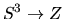where Z denotes the 6-skeleton of the Lie group G2. Thus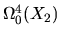is homotopy equivalent to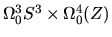.
(3)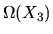is homotopy equivalent to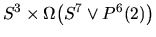.
(4)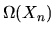is the homotopy theoretic fibre of a map from a finite complex to the Lie group Spin(n).

Further information concerning the map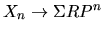is given. For example, the theorem above for X2 gives a different proof of a theorem of Jie Wu concerning a splitting of the homotopy groups for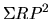.Next: Gustavo Granja - On HPn Up: 2)  Homotopy Theory / Théorie Previous: Dan Christensen - Phantom